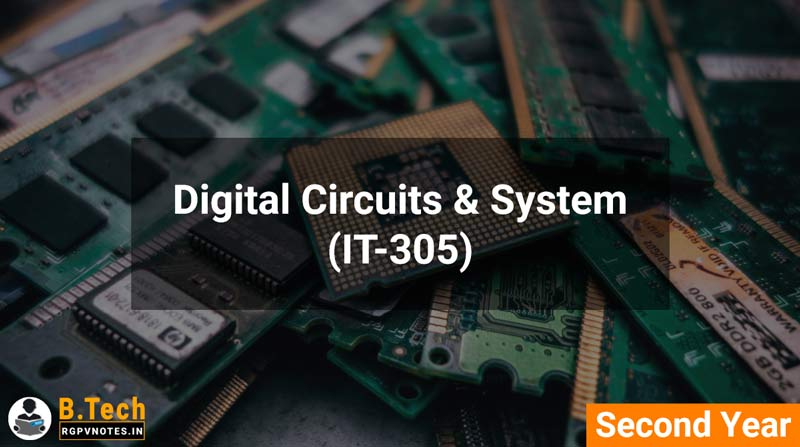# Digital Circuits & System (IT-305)## Course Objectives

1 Understand working of logic gates.
2 To design and implement combinational and sequential logic circuits
3 Understand the process of analog to digital and digital to analog conversion
4 To understand various logic families

## Syllabus

UNIT 1:
Number systems and logic gates: Decimal, Binary, Octal, Hexadecimal number systems and radix conversion. Codes- BCD, excess 3, gray, ASCII. Boolean algebra- Theorems and properties, Boolean functions, canonical and standard forms, De Morgans theorem, digital logic gates, Karnaugh maps.

UNIT 2:
Combinational circuits: Introduction to combinational circuits, multilevel NAND, NOR implementation. Designing binary Adders and Subtractors. Decoder, Encoder, Multiplexer, Demultiplexer circuits.

UNIT 3:
Sequential circuits: Introduction to Sequential circuits, flip-flops, RS, D, T, JK, M/S JK-flipflops, truth tables, excitation tables and characteristic equations, clocked and edge triggered flipflops, Registers- Definition, serial, parallel, shift left/right registers, Johnson counter, asynchronous and synchronous counters.

UNIT 4:
Digital logic families: Bipolar and unipolar logic families, Digital IC specifications, RTL, DTL, All types of TTL circuits, ECL, IIL, PMOS, NMOS & CMOS Logic

UNIT 5:
Clocks and timing circuits: Bistable, Monostable & Astable multivibrator, Schmitt trigger circuit, Introduction of Analog to Digital & Digital to Analog converters, Display devices, 7 and 16 segment LED display, LCD.

• Unit 1
• Unit 2
• Unit 3
• Unit 4
• Unit 5

## Course Outcomes

On the completion of this course 1 Students will be able to perform number base conversions, use Boolean logic to create digital circuits.
2. Student can understand use of encoders, decoders, multiplexers and demultiplexers in communication systems.
3 By learning design of combinational and sequential circuits student can understand its use in digital systems such as computers, communication systems and other modern technologies.
4 Study of ADC and DAC along with display devices will enable students to understand signal conversion and its display and their applications in digital devices.

## Books Recommended

1. M. Morris Mono, “Digital logic design”, Pearson Education Pvt. Ltd.
2. A Anand Kumar, “Fundamentals of digital circuits“, PHI Learning Pvt Ltd.
3. A K Maini, “Digital Electronics Principles and Integrated Circuits, Wiley India Pvt Ltd.
4. R P Jain, “Modern Digital Electronics”, Tata McGraw-Hill publishing company Ltd.
5. D P Kothari and J S Dhillon, "Digital Circuits and Design", Pearson Education Pvt. Ltd.

## List of Experiments:

1. Study and verify the operation of AND, OR, NOT, NOR and NAND logic gates.
2. Design all basic logic gates using NOR universal gate.
3. Design all basic logic gates using NAND universal gate.
4. Verification of Demorgan’s theorem.
6. Construction and verification of half subtractor and full subtractor circuits.
7. Design of Binary to Grey & Grey to Binary code Converters .
8. Design of BCD to excess-3 code converter.
9. Design and verification of Multiplexer circuit
10. Design and verification of De-multiplexer circuit.

## You May Also Like

Contact author here.